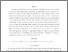# Distortion Outage Minimization and Diversity Order Analysis for Coherent Multi-Access

Wang, Chih-Hong and Leong, Alex S. and Dey, Subhrakanti (2011) Distortion Outage Minimization and Diversity Order Analysis for Coherent Multi-Access. IEEE Transactions on Signal Processing, 59 (12). pp. 6144-6159. ISSN 1053-587XPreviewmore...Add this article to your Mendeley library

## Abstract

In this paper we investigate the distortion outage performance of distributed estimation schemes in wireless sensor networks, where a distortion outage is deﬁned as the event that the estimation error or distortion exceeds a pre-determined threshold. The sensors transmit their observation signals using the analog amplify and forward scheme through coherent multi-access channels to the fusion center, which reconstructs a minimum mean squared error (MMSE) estimate of the physical quantity observed. We consider three power allocation schemes - 1) equal power allocation (EPA), 2) short-term optimal power allocation (ST-OPA), where we minimize the distortion subject to a power constraint at each time step, and 3) long-term optimal power allocation (LT-OPA), where we minimize the distortion outage probability subject to a long-term average power constraint. We study their diversity orders of distortion outage in terms of increasing numbers of sensors, and show that under Rayleigh fading EPA and ST-OPA achieve the same diversity order of N logN, where N is the number of sensors. This suggests that in the case of a large number of sensors, the spatial diversity gain in EPA can overcome fading equally well as in ST-OPA. On the other hand, in LT-OPA, we ﬁnd that for N > 1 the outage probability can be driven to zero with a ﬁnite amount of total power.

Item Type: Article Distortion; Outage; Minimization; Diversity; Analysis; Coherent Multi-Access; Faculty of Science and Engineering > Electronic Engineering 12713 https://doi.org/10.1109/TSP.2011.2165950 Subhrakanti Dey 07 Apr 2020 09:05 IEEE Transactions on Signal Processing Institute of Electrical and Electronics Engineers YesItem control page